Next: 3.1.1 Evaluation of Element Up: 3. Numerical Discretization Methods Previous: 3. Numerical Discretization Methods

# 3.1 Finite Elements

The method of Galerkin's weighted residuals [Sch80][Zie89][Oña91] is used as a general formulation for the discretization of partial differential equations even if an extreme formulation can not be found as for example in case of Ritz's approach [Sch80][Sch97b] .

In Galerkin's approach the basic equation is weighted with appropriate discrete weighting functions and the resulting equation integrated over the region of interest is equaled to zero.

For the discretization of the Laplace operator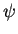== 0 (3.1)

whereis a potential distribution, the application of the weighted residual approach results in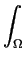wi()d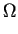= 0                i = 1, 2,..., n (3.2)

where wi denotes a weighting function associated with a discrete point i within a given discretized domain with n discrete points.

To solve (3.2) a spatial variation ofmust be found. The first step is to subdivide the simulation domain into a number of subdomains called elements (Fig. 3.1). Each element is associated with a number of discrete points or nodes located within the element or on its boundary. The spatial variation ofwithin an element is then defined in terms of the values at the belonging node points. If the element has n node points with associated values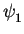,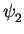,  ...  ,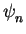the value ofcan be formulated as=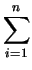Ni .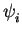(3.3)

where N is a function of the spatial coordinates of the n element points. These polynomials are usually referred to as shape functions.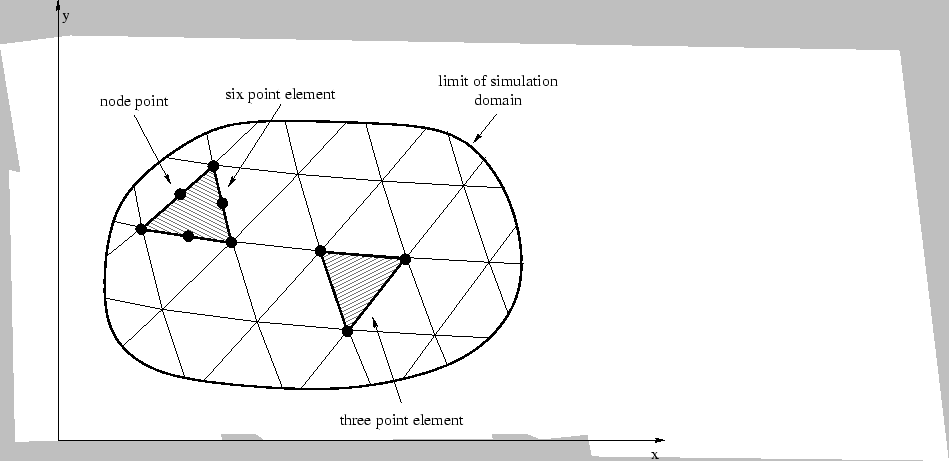Having completed a spatial subdivision of the simulation domain and after allocation of suitable shape functions, the next step is to integrate (3.2) over each element. This is usually accomplished by some form of numerical integration (see also Chapter 3.1.2) leading to an element contribution of the form

 h .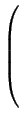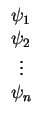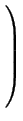(3.4)

with h denoting the element matrix.

All element contributions are summed up and the resulting final system matrix H

 H .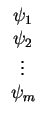= 0 (3.5)

is found, where m is the total number of nodal points in the simulation domain. After definition of appropriate boundary conditions the matrix equation (3.5) has to be solved for the nodal values of the desired variables.Next: 3.1.1 Evaluation of Element Up: 3. Numerical Discretization Methods Previous: 3. Numerical Discretization Methods